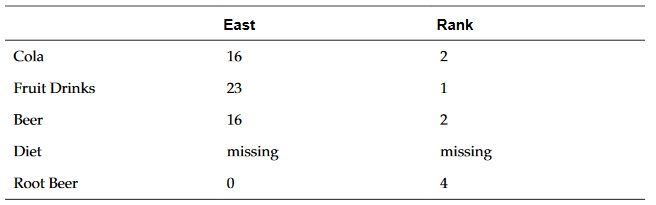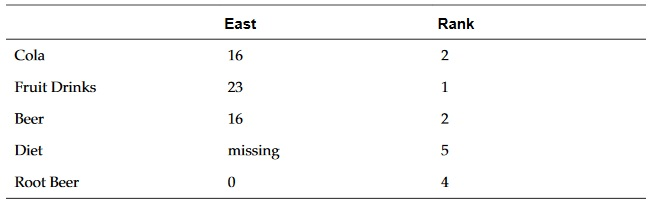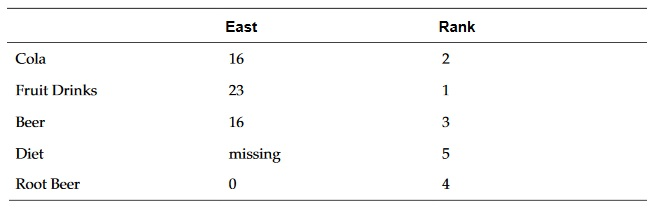## Rank

`Rank` is a financial function that provides a rank value for a value in a specified range. `Rank` is processed by Financial Reporting and does not depend on the database connection.

Syntax:

``` Rank([Reference], Order)```

``` Rank([Reference], Order, Unique)```

Argument Description

Reference

The range of cells, rows, or columns to rank, with letters identifying columns and numbers identifying rows. For example, specify [A,1:5] to rank the values for rows 1 through 5 in column A.

You can use the .ifNN property with a range of cells to assign numbers to any cells with nonnumeric values so that those cells can be ranked. For example, you can use .ifNN(-1) to assign the value -1 to any cell with a missing value.

Order

Indicates the order by which the values are ranked. The lowest value ranked in ascending order receives a rank result of 1. The largest value ranked in descending order receives a rank result of 1. The order can be indicated by any of the following keywords or values:

• Ascending

• Descending

• Asc

• Des

• Desc

• 1 (the number 1 is the same as Ascending)

• 0 (zero is the same as Descending)

The keywords are not case-sensitive.

Do not enclose the number or keyword indicating order in quotation marks.

Unique

(Optional) A Boolean keyword indicating how to treat equal values in the Reference parameter where:

• false (or omitted) — equal values receive the same ranking ; ranked results may be duplicated

• true — equal values receive a unique ranking; there are no duplicate rankings. Values in the Reference parameter are ranked on a first come, first ranked basis. For example, if values in rows 2 and 5 are equal, the value in row 2 is ranked before that of row 5.

Examples:

This formula in column B ranks the values in rows 1 through 5 in column A in descending order:

``Rank([A,1:5], descending)``

The result might be as follows:When two values are equal, they receive the same rank value. In the example above, Cola and Beer have the same value and therefore the same rank.

This formula in column B assigns the value of -1 to any nonnumeric value so it can be ranked:

``Rank([A,1:5].ifNN(-1), descending)``

In the following result, the missing value now has a rank of 5:Example:

The following example builds on the previous example explaining how the new "unique" parameter affects the results:

This formula in column B assigns the value of -1 to any nonnumeric value so it can be ranked, and also indicates that each ranking should be unique:

``Rank([A,1:5].ifNN(-1), descending, true)``

In the following result, the missing value now has a rank of 5, and Beer has a value of 3 (even though it has the same data value as Cola):